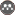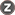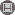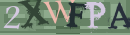Volume 17, Issue 40 (Mathematic- 2015)                   2015, 17(40): 63-74 | Back to browse issues page

BibTeX | RIS | EndNote | Medlars | ProCite | Reference Manager | RefWorks
Send citation to:Mir Mehrabi E. The stability of non-standard finite difference scheme for solution of partial differential equations of fractional order. Quarterly Journal of Science Kharazmi University. 2015; 17 (40) :63-74
URL: http://jsci.khu.ac.ir/article-1-2032-en.html
Abstract:   (5332 Views)
Fractional derivatives and integrals are new concepts of derivatives and integrals of arbitrary order. Partial differential equations whose derivatives can be of fractional order, are called fractional partial differential equations (FPDEs). Recently, these equations have been under special attentions due to their most practical usages. In this paper, we survey a rather general case of FPDE, to obtain a numerical scheme, the fractional derivatives in the equation are replaced by common definitions such as Grundwald-Letnikov, Riemann-Liouville and Caputo, to improve the numerical solution, partial derivatives inside the equation are discrete using non-standard finite difference scheme. Then, we survey the stability of numerical scheme and prove that the proposed method is unconditionally stable. Eventually, in order to approve the theoretical results, we use presented technique to solve wave equation with fractional-order that is very practical and widely used in physics and its branches. Numerical results confirm the findings of the theory and show that this technique is effective.Rights and permissionsThis work is licensed under a Creative Commons Attribution-NonCommercial 4.0 International License.